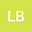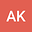On the Stefan Problem With Nonlinear Thermal Conductivity
••Ammar Khanfer
Prince Sultan University
Author Profile## Abstract

The solution is obtained and validated by an existence and uniqueness theorem for the following nonlinear boundary value problem $\frac{d}{dx}(1+\delta y+\gamma y^{2})^{n}\frac{dy}{dx}]+2x\frac{dy}{dx}=0,\,\,\,x>0,\,\,y(0)=0,\,\,\,y(\infty)=1,$ which was proposed in 1974 by  to represent a Stefan problem with a nonlinear temperature-dependent thermal conductivity on the semi-infinite line (0;1). The modified error function of two parameters $\varphi_{\delta,\gamma}$ is introduced to represent the solution of the problem above, and some properties of the function are established. This generalizes the results obtained in [3, 4].
06 Sep 2021Submitted to Mathematical Methods in the Applied Sciences
07 Sep 2021Submission Checks Completed
07 Sep 2021Assigned to Editor
12 Sep 2021Reviewer(s) Assigned
04 Feb 2022Review(s) Completed, Editorial Evaluation Pending
05 Feb 2022Editorial Decision: Revise Major